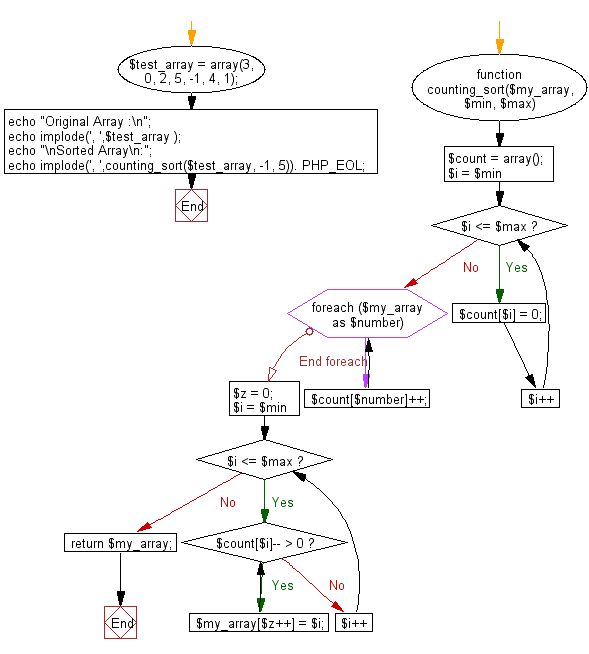﻿ PHP: Sort a list of elements using Counting sort - w3resource

# PHP Searching and Sorting Algorithm: Counting sort

## PHP Searching and Sorting Algorithm: Exercise-11 with Solution

Write a PHP program to sort a list of elements using Counting sort.

According to Wikipedia "In computer science, counting sort is an algorithm for sorting a collection of objects according to keys that are small integers; that is, it is an integer sorting algorithm. It operates by counting the number of objects that have each distinct key value, and using arithmetic on those counts to determine the positions of each key value in the output sequence. Its running time is linear in the number of items and the difference between the maximum and minimum key values, so it is only suitable for direct use in situations where the variation in keys is not significantly greater than the number of items. However, it is often used as a subroutine in another sorting algorithm, radix sort, that can handle larger keys more efficiently".

The algorithm loops over the items, computing a histogram of the number of times each key occurs within the input collection. It then performs a prefix sum computation (a second loop, over the range of possible keys) to determine, for each key, the starting position in the output array of the items having that key. Finally, it loops over the items again, moving each item into its sorted position in the output array.

Sample Solution :

PHP Code :

``````<?php
function counting_sort(\$my_array, \$min, \$max)
{
\$count = array();
for(\$i = \$min; \$i <= \$max; \$i++)
{
\$count[\$i] = 0;
}

foreach(\$my_array as \$number)
{
\$count[\$number]++;
}
\$z = 0;
for(\$i = \$min; \$i <= \$max; \$i++) {
while( \$count[\$i]-- > 0 ) {
\$my_array[\$z++] = \$i;
}
}
return \$my_array;
}
\$test_array = array(3, 0, 2, 5, -1, 4, 1);
echo "Original Array :\n";
echo implode(', ',\$test_array );
echo "\nSorted Array\n:";
echo implode(', ',counting_sort(\$test_array, -1, 5)). PHP_EOL;
?>
```
```

Sample Output:

```Original Array :
3, 0, 2, 5, -1, 4, 1
Sorted Array :
-1, 0, 1, 2, 3, 4, 5
```

Flowchart :PHP Code Editor:

Have another way to solve this solution? Contribute your code (and comments) through Disqus.

What is the difficulty level of this exercise?

Test your Programming skills with w3resource's quiz.

﻿